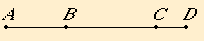# Proposition 83

To a straight line which produces with a rational area a medial whole only one straight line can be annexed which is incommensurable in square with the whole straight line and which with the whole straight line makes the sum of squares on them medial but twice the rectangle contained by them rational.
X.77

Let AB be the straight line which produces with a rational area a medial whole, and let BC be an annex to AB. Then AC and CB are straight lines incommensurable in square which fulfill the given conditions.

I say that no other straight line can be annexed to AB which fulfills the same conditions.X.77

If possible, let BD be so annexed. Then AD and DB are both straight lines incommensurable in square which fulfill the given conditions.

X.26

As in the preceding cases, the excess of the sum of the squares on AD and DB over the sum of the squares on AC and CB is also the excess of twice the rectangle AD by DB over twice the rectangle AC by CB, while twice the rectangle AD by DB exceeds twice the rectangle AC by CB by a rational area, for both are rational, therefore the sum of the squares on AD and DB also exceeds the sum of the squares on AC and CB by a rational area, which is impossible, for both are medial.

Therefore no other straight line can be annexed to AB which is incommensurable in square with the whole and which with the whole fulfills the aforesaid conditions, therefore only one straight line can be so annexed.

Q.E.D.

## Guide

This proposition is not used in the rest of the Elements.